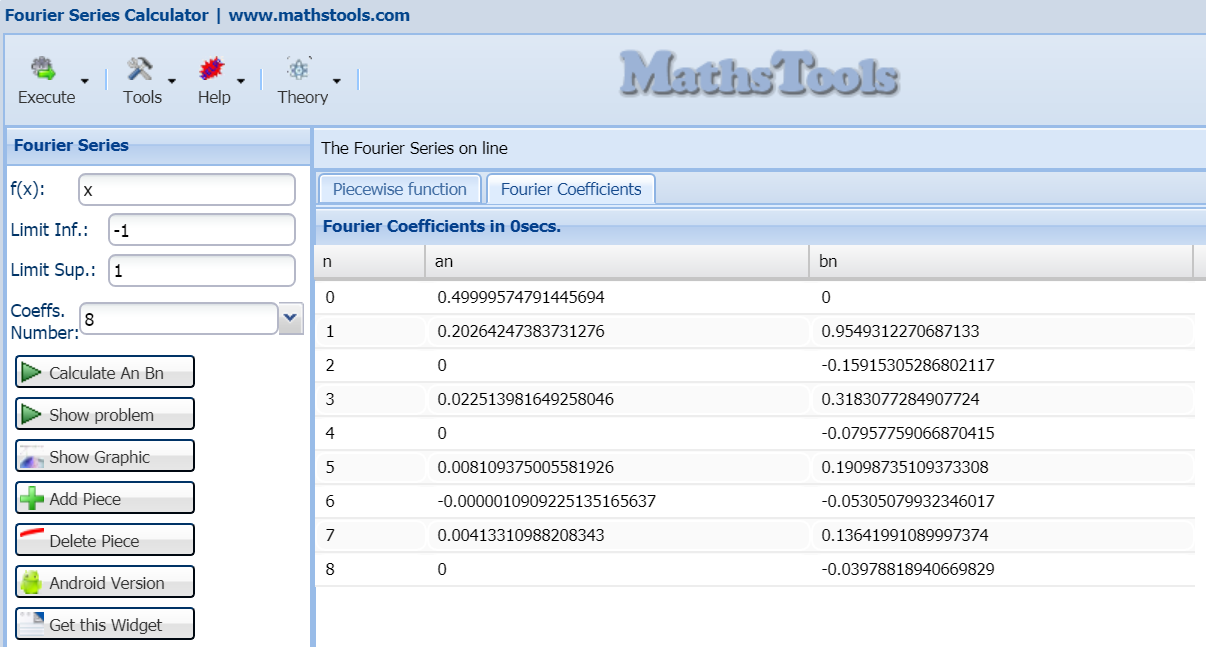# Fourier coefficients and series

### Fourier series calculation example

Due to numerous requests on the web, we will make an example of calculation of the Fourier series of a piecewise defined function from an exercise submitted by one of our readers. The calculations are more laborious than difficult, but let's get on with it ...

It is asked to calculate the Fourier series of following picewise function

$f(x)=\left\{\begin{matrix} x & si\; x \in [-1,0)\\ 1 & si\; x \in [0, 1] \\ 0 & si\; |x|>1 \end{matrix}\right.$

The first thing of all is to remember that our formulas here seen refer to interval $[-\pi, \pi]$, so we perform a change of variable

$t = \pi x$ to obtain

$f(t)=\left\{\begin{matrix} \frac{t}{\pi} & si\; t \in [-\pi,0)\\ 1 & si\; t \in [0, \pi] \\ 0 & si\; |t|>\pi \end{matrix}\right.$

We can already apply our formulas for the Fourier series that we remember was

$$f(x)=\frac{A_{0}}{2} + \sum_{n=1}^{\infty}(A_n cos nx + B_nsin nx )$$ with $A_i, B_i \in \mathbb{R}$ and

(1)       $A_0= \frac{1}{\pi} \int_{-\pi}^{\pi}f(x) dx$

(2)      $A_n= \frac{1}{\pi} \int_{-\pi}^{\pi}f(x) cos nx dx$

(3)      $B_n= \frac{1}{\pi} \int_{-\pi}^{\pi}f(x) sin nx dx$

We start by calculating (1)

$A_0=\frac{1}{\pi}\int_{-\pi}^{0} \frac{t}{\pi}dt + \frac{1}{\pi}\int_{0}^{\pi} dt = \frac{1}{\pi}( \pi-\frac{\pi}{2}) = \frac{1}{2}$

The next, we calculate (2), for this our integral is separated into two pieces

$A_n=\frac{1}{\pi}\int_{-\pi}^{0} \frac{t}{\pi} cos(nt)dt + \frac{1}{\pi}\int_{0}^{\pi} cos(nt)dt = I_1 + I_2$

We start by calculating the first of the integrals I1, we do it by parts

$\left\{\begin{matrix} u= \frac{t}{\pi} \Rightarrow du=\frac{dt}{\pi} \\ dv= cos(nt) dt \Rightarrow v=\frac{sin(nt)}{n} \end{matrix}\right.$

Applying the method of integration by parts, whose formula we remember was

$\int udv = uv-\int vdu$

So

$I_1 = 0 - \frac{1}{\pi^2} \int_{-\pi}^{0} \frac{sin(nt)}{n}dt = ... = \frac{1}{(\pi n)^2} (1-(-1)^n)$

Now we calculate the second integral, we see that

$I_2 = 0$

$A_n= \frac{1}{(\pi n)^2} (1-(-1)^n)$

Patience, it only remains to calculate the Bn, it is very similar to the previous calculation

$b_n=\frac{1}{\pi}\int_{-\pi}^{0} \frac{t}{\pi} sin(nt)dt + \frac{1}{\pi}\int_{0}^{\pi} sin(nt)dt = J_1 + J_2$

We start by calculating J1, we proceed as before by parts to obtain.

$J_1 = -\frac{(-1)^n}{n\pi}$

J2 is calculated directly

$J_2 = \frac{1}{n \pi}(1- (-1)^n)$

Therefore

$B_n = \frac{2-(-1)^n}{n\pi}$

We already have all the ingredients for the Fourier series formula, it is as follows

$f(t)=\frac{1}{4}+\sum_{n=1}^{\infty}\frac{1}{(\pi n)^2} (1-(-1)^n)cos(nt) + (\frac{2-(-1)^n}{n\pi}) sin(nt)$

Almost everything is done, the only thing now is to undo the initial variable change to finally get:

$f(x)=\frac{1}{4}+\sum_{n=1}^{\infty}\frac{1}{(\pi n)^2} (1-(-1)^n)cos(n \pi x) + (\frac{2-(-1)^n}{n\pi}) sin(n \pi x)$

To test our result we can go to Fourier Series calculator on this same site, if we introduce our function it remainsif we click on calculate, with 8 coefficients we obtainwe see that numerical results coincide (except truncation error) with the obtained values, the graph of Fourier series remains## Was useful? want add anything?

Post here

### Post from other users

Post here
Update cookies preferences